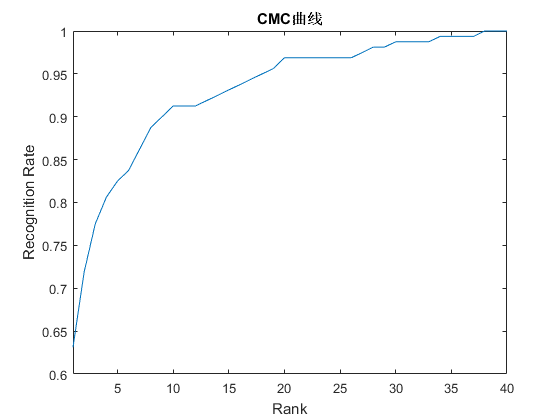# 模式识别分类器评价指标之CMC曲线

CMC曲线全称是Cumulative Match Characteristic (CMC) curve，也就是累积匹配曲线，同ROC曲线Receiver Operating Characteristic (ROC) curve一样，是模式识别系统，如人脸，指纹，虹膜等的重要评价指标，尤其是在生物特征识别系统中，一般同ROC曲线一起给出，能够综合评价出算法的好坏。如下图所示：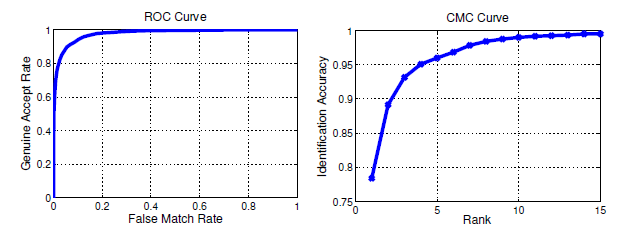# CMC曲线原理

CMC曲线综合反映了分类器的性能，它的评价指标与现在deep learning中常用的top1 err或top5 err评价指标一样的意思，不同的是这里Rank1 recognition rate表示的是正确率而不是错误率，两者的关系是

Rank1识别率=1-top1 err

Rank5识别率=1-top5 err

# Matlab编程实现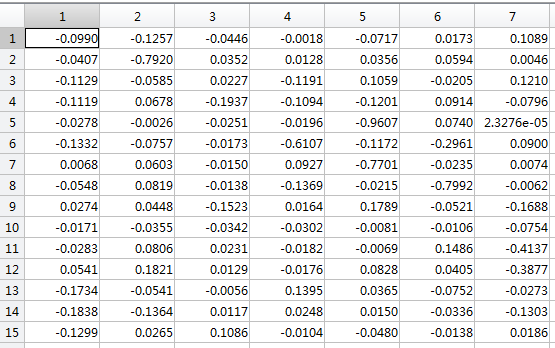%处理测试样本与注册的训练样本匹配的相似度矩阵
match_scores = zeros(length(num_test),length(num_class));
true_labels = zeros(length(num_test),length(num_class));
for i=1:length(num_test)
for j=1:length(num_class)
[x,y]=find(num_class(j)==num_train);

%选取匹配程度的中值
label_distances(i,j) = median(match_dist(i,y));
if num_test(i)==num_class(j)
true_labels(i,j)=1;
end
end
end

%生成CMC
max_rank = length(num_class);

%Rank取值范围
ranks = 1:max_rank;

%排序
label_distances_sort = zeros(length(num_test),length(num_class));
true_labels_sort = zeros(length(num_test),length(num_class));
for i=1:length(num_test)
[label_distances_sort(i,:), ind] = sort(label_distances(i,:));
true_labels_sort(i,:) =  true_labels(i,ind);
end

%迭代
rec_rates = zeros(1,max_rank);
tmp = 0;
for i=1:max_rank
tmp = tmp + sum(true_labels_sort(:,i));
rec_rates(1,i)=tmp/length(num_test);
end  

num_test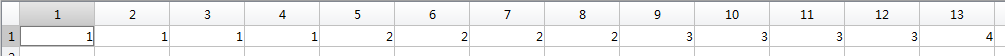num_class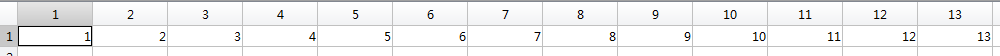num_train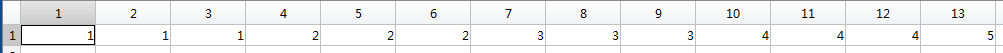plot(1:max_rank,rec_rates)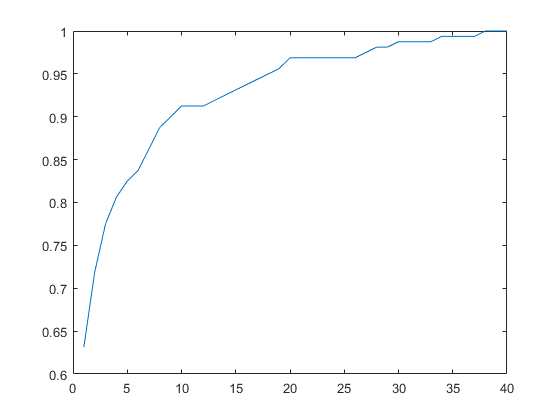hold on
set(gca,'XLim',[1 40]);%X轴的数据显示范围
set(gca,'YLim',[0.6 1]);%X轴的数据显示范围
%set(gca,'XTickLabel',[1:5:40]);%给坐标加标签
title('CMC曲线')
xlabel('Rank')
ylabel('Recognition Rate')